• Call Now

1800-102-2727•

# Reflection of waves, practice problem, FAQs

When you throw a ball towards the wall, it collides with the wall and returns to you. Do you think? What happens when a wave collides with the wall? If a wave collides with the wall it also bounces back with some changes. If the wave collides with a rigid boundary its phase changes.If the wave collides with a flexible boundary it will be in the same phase.This is called reflection of wave. Let's see this in detail !

Table of content

## Reflection of a String Wave from a Fixed End

Consider a wave pulse moving along the string from the free end to the other end of the string which is fixed to a wall, in the positive x-direction and getting reflected. Assume that there is no transmission of waves into the denser medium.When the pulse reaches the fixed end of the string, the string applies an upward force on the support. By Newton’s third law, the support exerts equal and opposite force on the pulse. Hence, the pulse inverts upon reflection.

Lets the equation of incident wave is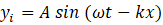Then After the reflection, the wave travels in a negative x-direction.So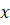will be replace by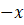and the equation of the wave becomes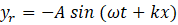The negative sign in the expression of the reflected wave suggests the phase change of 180° (or it is also due to the negative amplitude of the reflected wave).

## Reflection of a String Wave from a Free End

The free end of the string is connected to the massless ring on frictionless support as shown in the figure.When the traveling pulse reaches the free end of the string, it is free to move up and down about the frictionless support, the wave pulse exerts force on the free end of the string, causing upward acceleration of the ring. Then, the downward component of the tension pulls the ring back down. Here, the reflected pulse will be in the same phase.

After the reflection, the wave travels in a negative x-direction. So, the required equations of the waves are follows: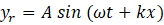The change of phase is zero when the end is free.

## Reflection of a String Wave from a partially fixed and partially Free End

• The wave pulse travels from a rarer medium to a denser medium

Suppose that a light string of linear mass density μ1 is attached to a heavy string of linear mass density μ2. Since the velocity of the wave in a medium is defined as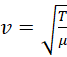, and here, the tension is same for both the strings and μ1 < μ2, Therefore v1 > v2. So, the light string can be considered as the rarer medium and the heavy string can be considered as the denser medium.This is the case where the boundary between the two strings is neither completely fixed nor completely free. Hence, a partial reflection takes place along with a partial transmission.

When the wave pulse reaches the boundary, there will be a reflection in the medium 1 and transmission in the medium 2. For a wave pulse that is traveling from the rarer medium to the denser medium at the boundary, there will be a phase change of 180° for the reflected wave.

And the transmitted wave will be in the same phase.As shown in figure,The velocity of the reflected wave remains the same, as it is reflected back to the same medium, i.e.,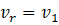. For the transmitted wave pulse,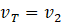.

The respective wave equations for reflection and transmission are as follows: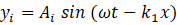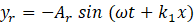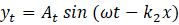• The wave pulse travels from a denser medium to a rarer medium

As discussed above, there will be transmission and reflection of the wave at the boundary in this case as well.When a pulse that is traveling on a heavy string reaches the light string, both reflected and transmitted wave pulses are the same. Hence, there will not be any phase change.The velocity of the wave pulse will increase as it is going from a denser to rarer medium.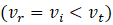.The respective wave equations for reflection and transmission are as follows: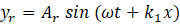## Practice problem

Q1. A pulse shown here is reflected from a rigid wall, A, and then from the free end, B. What will be the shape of the string after these two reflections?Answer:  At a fixed end, the wave is traveling from the rarer medium to the denser medium. This means that there will be a phase change of π. Now, after getting reflected from wall A, it travels towards free end B in an inverted shape. At the free end, the wave is traveling from the denser medium to the rarer medium (massless ring), i.e., no phase change occurs. Hence, the resultant wave will be as follows:Q2. The equation of a wave traveling in string is given by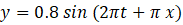. If the wave is reflected from a rigid boundary, then what is the equation of reflected wave?We can see from the equation the incident wave is traveling along -ve x-direction, After the reflection wave will travel along +ve x-direction. As the reflection is from Rigid boundary a phase change of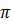will be there.

So the reflected wave is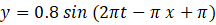Or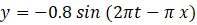Q3. A wave is traveling along the string of linear mass density 0.25 kg/m, it is transmitted to a second string of linear mass density 1 kg/m. If the velocity of the wave in the first string is 9 m/s, find the wave speed after transmission .
Answer:   Given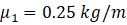and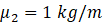,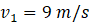Velocity of wave in first string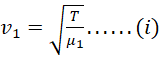Velocity of wave in second string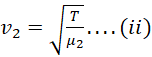Dividing eq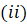from eq.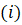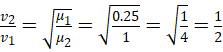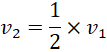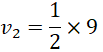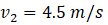Ans

Q4. A wave is traveling along a heavy string having linear mass density 0.78 kg/m, it is reflected from a light string tied on the end of the string. If the velocity of the incident wave is 19 m/s, find the velocity of the reflected wave.
Answer:  As the reflected wave will also be the in heavy string so the velocity of reflected wave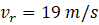.

## FAQs

Question 1. What is a denser medium?
Answer:   A medium is said to be denser relative to the other medium where the wave travels slower. glass is denser in comparison to water.

Question 2. What is a rarer medium?
Answer:  A medium is said to be rarer relative to the other where the wave travels faster. Air is rarer in comparison to water.

Q3. Is the sound wave also reflected?
Answer:  Yes, sound waves also reflect.

Q4. What is the effect on the amplitude of reflected and transmitted waves?
Answer:  As the power of the incident wave is distributed between reflected and transmitted waves, Hence Amplitude of reflected and transmitted waves is reduced as compared to amplitude of initial wave.Talk to our expert
Resend OTP Timer =
By submitting up, I agree to receive all the Whatsapp communication on my registered number and Aakash terms and conditions and privacy policy